# Universal highly arc transitive digraphs

 Importance: High ✭✭✭
 Author(s): Cameron, Peter J. Praeger, Cheryl E. Wormald, Nicholas C.
 Subject: Graph Theory » Infinite Graphs
 Keywords: arc transitive digraph
 Posted by: mdevos on: October 21st, 2007

An alternating walk in a digraph is a walk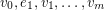so that the vertexis either the head of bothand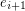or the tail of bothandfor every. A digraph is universal if for every pair of edges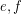, there is an alternating walk containing bothandQuestion   Does there exist a locally finite highly arc transitive digraph which is universal?

Letbe a digraph. For a nonnegative integer, a-arc inis a sequence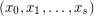of vertices so that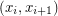is an edge for everyandfor every. We say thatis-arc transitive if its automorphism group acts transitively on the set ofarcs, and we say thatis highly arc transitive if it is-arc transitive for every. Note that the condition-arc transitive is precisely equivalent to vertex transitive.

It is an easy exercise to show that the only finite digraphs which are highly arc transitive are directed cycles. Since such graphs have only trivial alternating walks (only one edge can be used), they are not universal. Thus, any graph satisfying the criteria of the conjecture must be infinite.

Let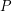be a two way infinite directed path (i.e. the Cayley graph onwith generating set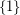). The digraphis not universal, but moreover, any digraph with a homomorphism ontocannot be universal. In the same article where the above question was posed, the authors asked wether there exist infinite highly transitive digraphs with no homomorphism onto. This question has since been resolved in the affirmative: Evans [E] constructed such a digraph with infinite indegree, and Malnic et. al. [MMSZ] have constructed a locally finite one.

In a vertex transitive digraph, every vertex must have the same indegree and the same outdegree, and we shall denote these by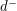and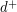respectively. A theorem of Praeger [P] shows that every locally finite highly transitive digraph for whichhas a homomorphism ontoand thus is not universal. More recently, Malnic et. al. [MMMSTZ] have established a condition on edge stabilizers in arc transitive digraphs which implies that any such digraph with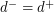a prime is not universal. It follows that any digraph satisfying the conditions of the highlighted question must havea composite number.

## Bibliography

*[CPW] P. J. Cameron, C. E. Praeger, and N. C. Wormald, Infinite highly arc transitive digraphs and universal covering digraphs. Combinatorica 13 (1993), no. 4, 377--396. MathSciNet.

[E] D. M. Evans, An infinite highly arc-transitive digraph, European J. Combin., 18 (1997) 281--286. MathSciNet.

[MMMSTZ] A. Malnic, D. Marusic, R. G. Moller, N. Seifter, V. Trofimov, and B. Zgrablic, Highly arc transitive digraphs: reachability, topological groups. European J. Combin. 26 (2005), no. 1, 19--28. MathSciNet.

[MMSZ] A. Malnic, D. Marusic, N. Seifter, and B. Zgrablic, Highly arc-transitive digraphs with no homomorphism onto Z. Combinatorica 22 (2002), no. 3, 435--443. MathSciNet

[P] C. E. Praeger, On homomorphic images of edge transitive directed graphs, Australas. J. Combin., 3 (1991), 207--210. MathSciNet.

* indicates original appearance(s) of problem.